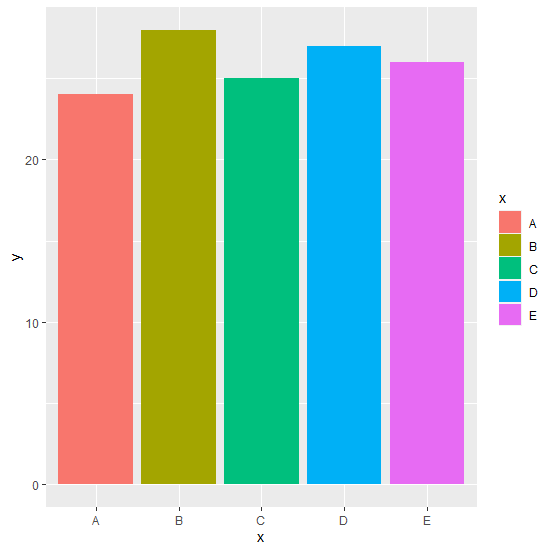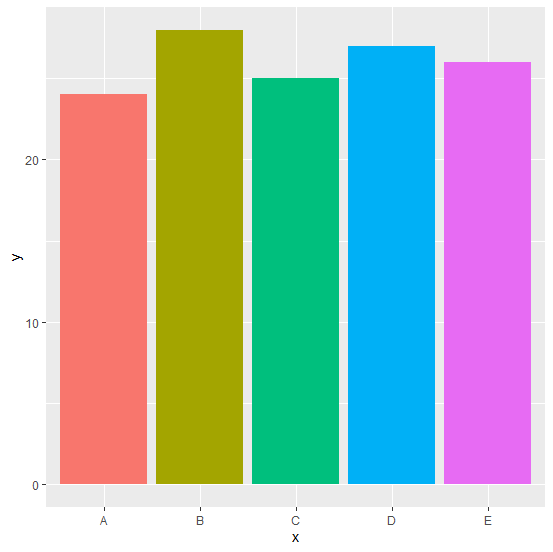# How to create colored barplot using ggplot2 without legend entries in R?

When we create a colored barplot using ggplot2 the legend entries are automatically created. If we want to create the plot without those legend entries then theme function can be used. For example, if we have a data frame df that contains x as categorical variable and y as count variable then barplot without legend entries can be created as:

ggplot(df,aes(x,y,fill=x))+geom_bar(stat="identity")+theme(legend.position="none")

## Example

Consider the below data frame:

Live Demo

> x<-c("A","B","C","D","E")
> y<-c(24,28,25,27,26)
> df<-data.frame(x,y)
> df

## Output

x y
1 A 24
2 B 28
3 C 25
4 D 27
5 E 26

> library(ggplot2)
> ggplot(df,aes(x,y,fill=x))+geom_bar(stat="identity")

## Output:Creating the barplot without legend entries:

> ggplot(df,aes(x,y,fill=x))+geom_bar(stat="identity")+theme(legend.position="none")

## Output: